Courses

# RD Sharma Solutions - Ex-5.1, Operations On Rational Numbers, Class 7, Math Class 7 Notes | EduRev

## Class 7 : RD Sharma Solutions - Ex-5.1, Operations On Rational Numbers, Class 7, Math Class 7 Notes | EduRev

The document RD Sharma Solutions - Ex-5.1, Operations On Rational Numbers, Class 7, Math Class 7 Notes | EduRev is a part of the Class 7 Course RD Sharma Solutions for Class 7 Mathematics.
All you need of Class 7 at this link: Class 7

Q1. Add the following rational numbers: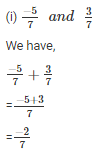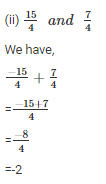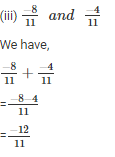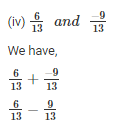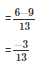Q2. Add the following rational numbers: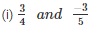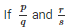are two rational numbers such that q and s do not have a common factor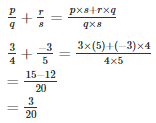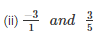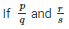are two rational numbers such that q and s do not have a common factor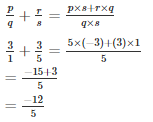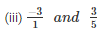LCM of 27 and 18 is 54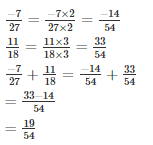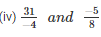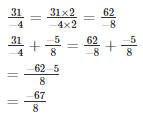Q3. Simplify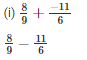LCM of 9 and 6 is 18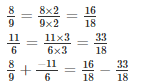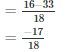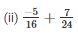LCM of 16 and 24 is 48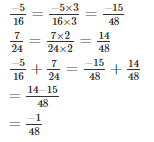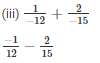LCM of 12 and 15 is 60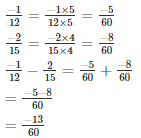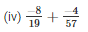LCM of 19 and 57 is 57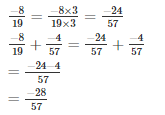Q4. Add and express the sum as a mixed fraction: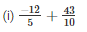LCM of 5 and 10 is 10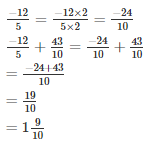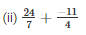LCM of 7 and 4 is 28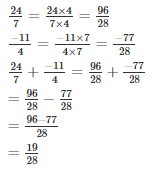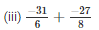LCM of 6 and 8 is 24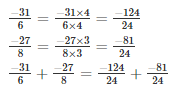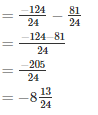Offer running on EduRev: Apply code STAYHOME200 to get INR 200 off on our premium plan EduRev Infinity!

97 docs

,

,

,

,

,

,

,

,

,

,

,

,

,

,

,

,

,

,

,

,

,

,

,

,

,

,

,

,

,

,

;